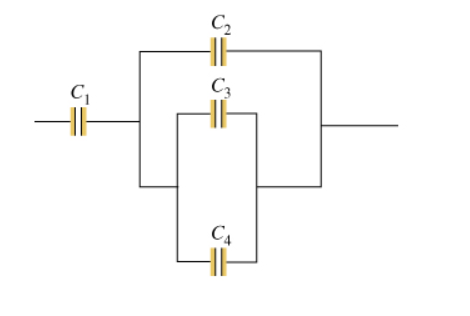# Problem: Consider the combination of capacitors shown in the diagram, where C1 = 3.00uF, C2 = 11.0 uF, C3 = 3.00 uF, and C4 = 5.00uF. Find the equivalent capacitance CA of the network of capacitors. Express your answer in microfarads.

###### FREE Expert Solution

For capacitors in parallel, the equivalent capacitance is:

$\overline{){{\mathbf{C}}}_{\mathbf{e}\mathbf{q}}{\mathbf{=}}{{\mathbf{C}}}_{{\mathbf{1}}}{\mathbf{+}}{{\mathbf{C}}}_{{\mathbf{2}}}{\mathbf{+}}{\mathbf{.}}{\mathbf{.}}{\mathbf{.}}{\mathbf{+}}{{\mathbf{C}}}_{{\mathbf{n}}}}$

For 2 capacitors in series, the equivalent capacitance is:

$\overline{){{\mathbf{C}}}_{\mathbf{e}\mathbf{q}}{\mathbf{=}}\frac{{\mathbf{C}}_{\mathbf{1}}{\mathbf{C}}_{\mathbf{2}}}{{\mathbf{C}}_{\mathbf{1}}\mathbf{+}{\mathbf{C}}_{\mathbf{2}}}}$

Capacitors C3 and C4 are in parallel.

79% (487 ratings)###### Problem Details

Consider the combination of capacitors shown in the diagram, where C1 = 3.00uF, C2 = 11.0 uF, C3 = 3.00 uF, and C4 = 5.00uF.